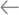# LearnTo All PostsBest Investment Calculator

17.10.2020

110

The investment calculator allows you to calculate the final amount that you will receive on a certain investment period, taking into account compound interest, when the accrued interest starts working for you and they also generate additional income. It will also help determine how much time you need to reach the required amount of capital and how much you need to invest in this. Otherwise, it can still be called a “money-saving calculator”.

The investment calculator will become your indispensable assistant in the case when you need to calculate the profit on the invested funds, considering the accumulating (complex) interest.

## Why do you need it?

The simplest example will answer this question: you decided to deposit money in a bank at interest and you want to calculate how much you will get back in 2 years, taking into account profit. Can you quickly do it on a regular calculator? The task is not simple, because every month the amount on which interest will accrue will increase. In addition to the calculator, you will need a pen, paper, and a considerable amount of time.

And now let’s see how long it takes to make the same calculations on the best investment calculator. All that you need to do is to enter information about the interest rate, the amount of the deposit, and the period for which you want to invest money. It took less than a second and you get the desired calculation!

If you plan to make additional investments (reinvestment) of funds every month, then this unit will help you out again. Fill in the field “Monthly fee” and at the exit, you will receive the amount of interest to you.

## What can it calculate?

### The best investment calculator will help to:

• Know the interest on the deposit without additional investments, but with capitalization;
• Determine the profitability of deposits with capitalization and regular replenishment;
• Learn the return on investment with compound interest and additional replenishment.

## How to use the calculator?

### The following fields are usually present in the calculator:

• Deposit — the initial amount that you deposit or invest.
• The number of periods — the period during which you plan to invest your money. The term can be specified both in years and months.
• Interest for the period — indicate the yield for 1 period.
• Regular investments — the amount you plan to invest additionally at the end of each period. If you do not plan to do this, leave the field blank.
• The currency.

### After filling in all the required fields, click on the “calculation” button and the calculator will give the result of:

• Your final amount at the end of the term, taking into account all capitalization and replenishment;
• The growth table of your investment for each period;
• The income you will receive at the end of the investment period

If you have already invested a certain amount that brings you a steady income and every month you withdraw a certain part, you can use the calculator to see how your savings will change over time.

The effect of compound interest really does wonders with your money. Try to fill different data in the fields and you will see how just a small change in the number in one of the fields can change the final amount and the resulting income at times!

### Example

If you invest 10,000 dollars for 15 years with a yield of 20% per annum, by the end of the term we will receive an amount of 154,070 dollars.

Now, make the same stuff, but for a period of 20 years. Then we already get 383 370, i.e. almost 2.5 times more.

If we invest an additional total of \$ 10,000 each, then our result will be \$ 2.24 million, which is almost 15 times more than the first example and 6 times more than the second.

## The best investment calculators

Use this list of the tools which FinanceWorld recommends to find your best investment calculator:

• CalcXML;
• Bankrate;
• Investment Loan;
• Vanguard’s Retirement Nest Egg ;
• Personal Capital’s Financial Dashboard, etc.

Here are only examples of these useful tools. Take time to find the best one for you yourself.

Subscribe
Notify of